Livio/ August 11, 2019/ Python/ 0 comments

Neural Networks

Neural Network are computer systems inspired by the human brain, which can ‘learn things’ by looking at examples. They can be used in tasks like image recognition, where we want our model to classify images of animals for example. At the end of the post we will use the class that we have built to make our computer recognize a number digit by looking at pictures. The main focus of this post is on building a class in Python that can do just that.

Model Representation

Neural networks are usually represented as below:The input layer corresponds to our training data, if for instance we’re trying to classify images, these would be a matrix m X n, where m is the number of images and n is the number of pixels of each image to which we would add a bias term (a column of 1’s) as we do in Logistic Regression.The output layer is how our training data should be classified. For instance, if we’re classifying digits from 0 to 9, this would be a m x 10 matrix (10 digits), where each column represents the probability of each image belonging to that category.

The hidden layers can be thought of as neurons which get switched on and off based on the activation function. They capture more and more complexity with every layer we add. They are the magic of neural networks and provide the discrimination necessary to be able to separate your training data. You can increase the number of neurons in a particular hidden layer or you can increase the number of hidden layers, or both. Increasing the number of neurons will allow you to decrease your training error but it also reduces the amount of generalization, which can be very important depending on your problem. This balance is something your learn to manage the more times you do it.

There are different activation functions that can be used, in this post we will the logistic function, which will look familiar: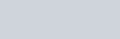Forward Propagation

The activation of the ‘neurons’ in our hidden layers is done by an algorithm called forward propagation which takes us from our input layer to our output layer. This is how it works visually:

image you have a neural network consisting of an input layer of 3 features, one hidden layer of 5 neurons, another hidden layer of 4 neurons, and an output layer of 3 classes. The network would be:Our input layer will be a m x 4 matrix, where m is the number of observations and 4 the number of features including the bias term (3+1)The step from the input layer to the first hidden layer is done by multiplying the input layer by our first thetas matrix, which is the first set of parameters our model will need to ‘learn’ in order to minimize the cost function I will show later:The multiplication gives us:on which we will calculate the logistic function and add a bias term, therefore our first hidden layer is equal to:The step from the first hidden layer to the second hidden layer is done by multiplying the input layer by our second thetas matrix:The multiplication gives us:on which we will calculate the logistic function and add a bias term, therefore our second hidden layer is equal to:The step from the second hidden layer to the output layer is done by multiplying the second hidden layer by our third thetas matrix:The multiplication gives us:on which we will calculate the logistic function:and so we have all our layers: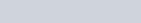Cost function

As with Logistic Regression, we are facing an optimization problem. Keeping as an example the neural network shown above, we need to find the thetas which minimize our cost function. The thetas are contained within the three thetas matrices shown above:It is important to notice that the first row of each theta matrix corresponds to the bias terms (they’re multiplied against the bias terms of their corresponding layer), this is an observation which will need to consider as we move along.

The cost function to minimize is: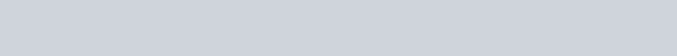Let’s look first at the part before the plus sign

This part is similar to that of the logistic regression, the only difference is that now we’re taking into account the errors on each class of Y, which is represented by the sum from k = 1 to p, where p is the number of classes in the output layer. If we are predicting just one class, like alive/dead, sick/not sick, then it would be exactly like the one of logistic regression. Imagine our training data is made up of just five rows, and we’ve a 3 classes classification problem, then our Y  matrix may look like this:If the first column was cat, the second column was a bird and the third column was a dog then this means that in our training data, the first image is of a cat, the second image is of a cat, the third image is of a bird, the fourth image is of a dog and the fifth image is of a bird.

Behind the scenes the first part of the cost function is doing (notice the element wise multiplication)+This results in a 5×3 matrix for which we need to sum all the elements and divide them by the number of samples (5) multiplied by -1, so -1/m.

The second part: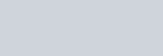is adding regularization. L represents the number of layers, in our example we’ve 4 layers and 3 thetas matrices, therefore the summation goes from h = 1 to 3 (L-1). Then we’re taking the square of all the thetas and summing them, except for those related to the bias terms (remember 1st row) therefore j goes from 2 (skips first row) to the size of the hth layer (Sh), and i goes from 1 to the size of the ith+1 layer(Sh+1). Lambda is our regularization parameter.

Back propagation

The goal is to find the values of thetas which minimize the cost function shown above. In order to do this, we will use the back propagation algorithm. Let’s consider again our neural network:We need to find the partial derivative of the cost function for each theta. In order to do this we will use the back propagation algorithm. Here is how it works:

Calculate the delta corresponding to the output layer, this is equal to: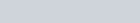this is a 5×3 matrix in our exampleOnce we have the delta, we are able to calculate the derivative of the cost function with respect to all the thetas belonging to the third thetas matrix: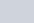which is: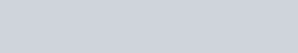Calculate the delta corresponding to the third layer: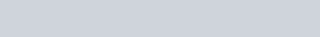this is a 5×5 matrix in our example

from which we remove the bias terms, which now correspond to the first column because we’ve transposed the thetas 3 matrix, so it becomes a 5×4 matrix

now we can calculate the derivative of the cost function with respect to the second thetas matrix:Calculate the delta corresponding to the second layer:this is a 5×6 matrix in our example

from which we remove the bias terms, which now correspond to the first column because we’ve transposed the thetas 2 matrix, so it becomes a 5×5 matrix

now we can calculate the derivative of the cost function with respect to the first thetas matrix:You can see the patterns:

The deltas, except for the one corresponding to the output layer, is equal to: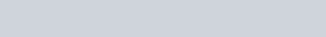and you remove the first column

And the partial derivatives: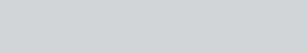What this algorithm is doing is propagating the error backwards from the output layer up until the first hidden layer, therefore our algorithm stops when we reach delta 2.

What our code will need to do is:Writing the Class

So, this is where the fun begins! Before starting to write our class, it is important to stop and think about how to implement it. The first parameters we need to pass to it are the number and sizes of each hidden layer, the value of the regularization parameter and the number of maximum iterations it should perform while minimizing the cost function. Therefore we have, the following __init__ method:

The second method we need to add is to calculate the logistic function:

The first thing our algorithm will need to do when the user trains the model is to create our thetas matrices. The size of each theta matrix is given by the sizes of each layer. A specific theta matrix l: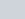has a number of rows equal to the size of l layer + 1: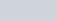and has a number of column equal to the size of the l+1 layer: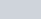Because the scipy.optimize algorithm needs a a vector of partial derivatives, we need to create a flattened version of our thetas matrices (all the thetas contained in a one dimensional array):into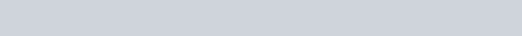The below method will create such flattened vector of thetas and also store the size of each theta matrix in order to recreate it when we vectorize the forward and back propagation steps:

we also need a method, as said, to reshape the flattened thetas into their appropriate sizes in order to take advantage of vectorized operations, the following method will do that for us:

So far, we have a way to create our flattened thetas, initialize them with random values and reshape them into appropriate matrices as needed. So we can take care of writing the forward propagation method, this will accept two parameters: X (a matrix corresponding to the input layer without the bias term which will be added by our method) and a list of thetas matrices properly shaped:

Now that we’ve a way to calculate each layer, we’re also ready to add the calculation of the cost function, which makes use of the above methods:

So we have our cost function and the forward propagation, we are just missing the gradient vector to feed into scipy.optimize function. But in order to have that, we need to implement back propagation first. Our back propagation algorithm will first calculate the delta corresponding to the output layer and then, in a backward loop, calculate all the remaining deltas based on how many layers we have and each time a delta is calculate, it also calculates the partial derivatives with respect the current thetas matrix:

now we’re able to create the gradient vector:

all the methods implement above are all ‘private’. When using the class, we will use the below ‘fit’ method to optimize the model:

The last method missing is a predict method:

Testing the Class

It is now time to test the model on real data. In this project we will categorize number digits from 0 to 9 by looking at images. The data set can be downloaded at the following links:

and are those used by Andrew Ng course: https://www.coursera.org/learn/machine-learning

The first file contains 5000 rows where each row represent a 20 pixel by 20 pixel grayscale image of the digit. Each pixel is represented by a floating point number indicating the grayscale intensity at that location. The pixels are unrolled into a 400-dimensional vector (20 x 20).

The second file contains 5000 rows and tells us how each image was classified. It contains 10 binary columns, where they represent the digits: 1, 2, 3, 4, 5, 6, 7, 8, 9, 0. For example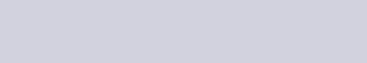tells us that first image of a 0, the second image of 4 and the third image of 6.

Below is a Jupyter Notebook example of how to use the class and the accuracy obtained from the dataset used. You can test different regularization parameters and number of iterations to see how this affect the predictions: